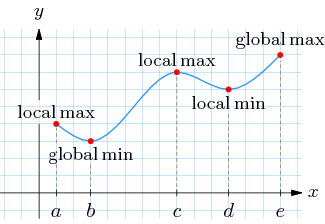# Extrema of a Function: Relative & Global

## What are the Extrema of a Function?Extrema of a Function are the maximums and minimums. They can be classified into two different types: global and local. Global extrema (also called absolute extrema) are the largest or smallest outputs of the function, when taken as a whole. Local extrema (also called relative extrema) are the largest or smallest values of a part of the function.

Contents:

## 1. Global Extrema (Absolute Extrema)

Global extrema are just the largest or smallest values of the entire function. In any function, there is only one global minimum (it’s the smallest possible value for the whole function) and one global maximum (it’s the largest value in the whole function).

You can find the global maximum by looking at a graph: look for the point where the “y” value is the highest. On the graph above, the highest point is at point e. In calculus, finding the extrema of a function is a little more complicated, and involves taking derivatives. See the following articles for step by step examples:

## Local Extrema (Relative Extrema)

Local extrema are the smallest or largest outputs of a small part of the function. For example, the function y = x2 goes to infinity, but you can take a small part of the function and find the local maxima or minima.

You can find the local extrema by looking at a graph. The local maximum will be the highest point on the graph between the specified range, while the local minimum will be the smallest value on the same range. Finding local extrema with calculus also involves taking the derivative of the function. See:

## References

Larson & Edwards, Calculus. Retrieved October 21, 2019 from: https://books.google.com/books?id=3-S5DQAAQBAJ.

CITE THIS AS:
Stephanie Glen. "Extrema of a Function: Relative & Global" From StatisticsHowTo.com: Elementary Statistics for the rest of us! https://www.statisticshowto.com/extrema-of-a-function-relative-global/